Next: What is simplex? Up: Convex Polyhedron Previous: What is the face   Contents

## What is a dual of a convex polytope?

For a convex polytope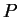, any convex polytope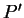withanti-isomorphic to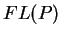(i.e. upside-down'' of) is called a (combinatorial) dual of. By the definition, a dual polytope has the same dimension as. The duality theorem states that every convex polytope admits a dual.

Theorem 1 (Duality of Polytopes)   Every nonempty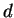-polytopein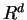admits a dual polytope in. In particular, one can construct a dual polytope by the following polar'' construction: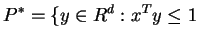for allwhereis assumed to contain the origin in its interior.

Whencontains the origin in its interior, the polytope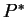is called the polar of. One can easily show that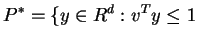for all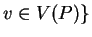where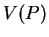denote the set of vertices of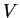, and this inequality (H-) representation ofis minimal (i.e. contains no redundant inequalities).Next: What is simplex? Up: Convex Polyhedron Previous: What is the face   Contents
Komei Fukuda 2004-08-26